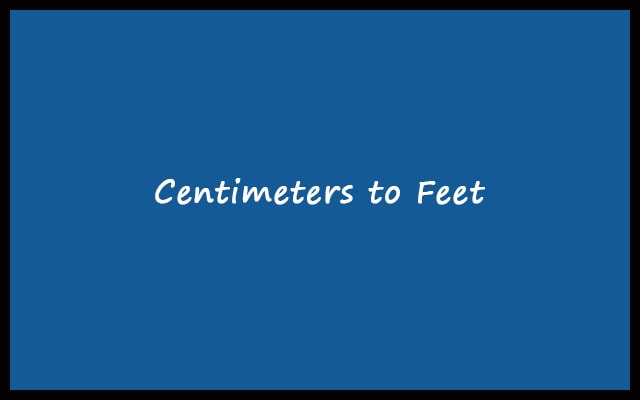# Cm to Feet – Centimeters to Feet Converter Calculator

Cm to Feet Converter will help you to convert Centimeters values into Feet. Also, the Centimeters to Feet conversion process is very easy and fast. Most importantly, this calculator is online. So, you can use it anytime and anywhere.

Formula: 1 cm = (1cm / 30.48) ft = 0.03280839895 ft
 Enter Centimeters: Feet Output: Feet + Inches Output: All Calculation:
Related## How to convert Centimeters to Feet / (cm to feet)?

As we know 1 centimeter is equal to 0.03280839895 feet.

So, the formula behind the calculation is:

#### 1 cm = (1cm / 30.48) ft = 0.03280839895 ft

Similarly, we can calculate feet values by divide the centimeter values with 30.48.

Therefore, the feet distance is equal to the centimeter distance divide by 30.48.

#### Feet (ft) = Centimeters (cm) / 30.48

In addition, Cm to Feet calculator also gives you the feet values with inches.

Now, let’s take some examples to understand it.

#### Example: 1

Convert 10 centimeters to feet:

So, ft = 10 cm / 30.48 = 0.3280839895 ft

#### Example: 2

Convert 150 centimeters to feet:

ft = 150 cm / 30.48 = 4.9212598425 ft

### How many centimeters in a foot?

Answer: There are 30.48 centimeters are in a foot.

### How many feet in a centimeter?

Answer: There are 0.03280839895 feet are in a centimeter.

#### How to use cm to feet converter?

It is very easy to use this converter. Just follow the simple steps.

1. Firstly, start the web browser and open the Centimeters to feet converter
2. Enter the centimeter value in text box.
3. Lastly, click on “Convert” button.
4. Also, you can reset all the field by clicking “Reset” button.

#### Centimeters to feet Conversion Table

Centimeters (cm) Feet (ft)
0.001 cm 0.0000328 ft
0.01 cm 0.000328 ft
0.1 cm 0.003281 ft
1 cm 0.032808 ft
2 cm 0.065617 ft
3 cm 0.098425 ft
4 cm 0.131234 ft
5 cm 0.164042 ft
6 cm 0.196850 ft
7 cm 0.229659 ft
8 cm 0.262467 ft
9 cm 0.295276 ft
10 cm 0.328084 ft
20 cm 0.656168 ft
30 cm 0.984252 ft
40 cm 1.312336 ft
50 cm 1.640420 ft
60 cm 1.968504 ft
70 cm 2.296588 ft
80 cm 2.624672 ft
90 cm 2.952756 ft
100 cm 3.280840 ft